## Friday, August 30, 2013

### Arduino: simple compass with HMC5883L + Library

#### Introduction

One of the most popular I2C-compatible magnetometer is the Honeywell HMC5883L. These sensors’ solid-state construction with very low cross-axis sensitivity is designed to measure both the direction and
the magnitude of Earth’s magnetic fields, from milli-gauss to 8  gauss.
In this tutorial I'll try to:
1. Introduce how a magnetometer works
2. Explain how to retrieve the heading from the magnetometer data
3. Provide the little library I wrote for Arduino IDE

#### How does a magnetometer work?

An electronic magnetometer like the HMC5883L is based on the Anisotropic Magnetoresistance phenomenon. Mastering the physics that descibe the phenomenon is not an easy task, since this is a huge field whose depths we cannot hope to begin to plumb in these few words.
Basically, the a magnetic field interacts with the path of the current flowing through a ferrous material, according to the Lorentz Law hence the resistance of the material seems to change to the observer. You can imagine as if the bar of ferrous material (e.g InSb) grows longer, raising its electric resistance. Therefore measuring the change in the resistance we can estimate the magnetical field! The Equation that rules the phenomenon is in the image below. For a further investigation of the matter, especially on the electronics a magnetometer is based upon, you could read this.

#### From the raw data to the north!Supercomputer models of Earth's magnetic field from nasa.gov

In a compass, the magnetic field you measure is the earth's one. It is tangential to the surface of the planet and it flows from north to south. The HMC5883L has three different axis to calculate the headings, as you may not know the tilt of your device (i.e. our quadcopter) when you need the data! Anyway for this example we will assume that the sensor is flat on a table, so we don't have to worry about its tilt. Therefore we'll use only X and Y axes data.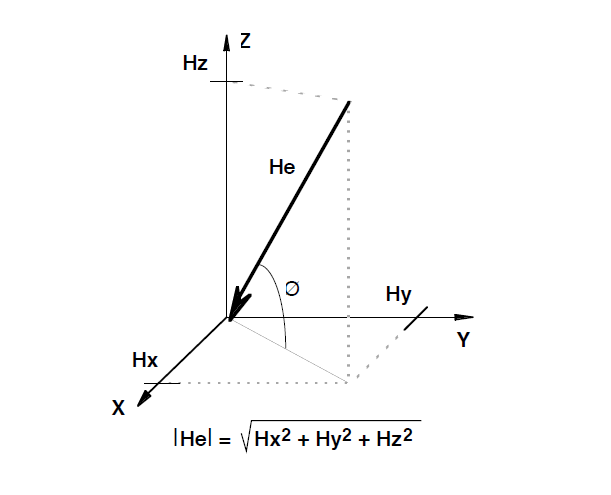We'll assume Hz =0

Hence the angle between the Y axis and the magnetic north will be, according to the quandrant:

Direction (y>0) = 90 - [arctan(x/y)] * 180 / π
Direction (y<0) = 270 - [arctan(x/y)] * 180 / π
Direction (y=0, x<0) = 180.0
Direction (y=0, x>0) = 0.0

First of all we have to scale the raw data according to the scale we chose.
The valid gauss values are: 0.88, 1.3, 1.9, 2.5, 4.0, 4.7, 5.6, 8.1. Of course for a geo-compass we just need 1.3 Ga, that leads us to a 0.92 [mG/LSb] of resolution and a gain of 1090 [LSb/Gauss]. The code I provide with this post is based on the code found here, but at the time this post is written, the original code won't work. There are some huge bugs as floating point number comparison that will not allow you to change the scale factor of the sensor, and some queer bugs on error handling (basically that code doesn't check for error at all, as you can easily prove executing it: it will always display an error setting the scale, and setting the measurement mode. More oddly this latter error display the same message because the error variable is not reset after its use). Of course even my library will have some bugs too, and it's not complete at all, but it's a good start to familiarize with the sensor itself.

Here is the code with a lots of comments:

On the image below you can see how the heading measured with an iPhone 4 is quite close to the one we read from Arduino. There are many margin of improvement. First, we ought compensate the potential tilt of the device using the accelerometer data from the ADXL345, for example using the info on my previous post! Moreover, my breadboard has an aluminium ground plane at its bottom, which can obviously make harder for the structure to sense the magnetic field and/or could drift it and create an offset.

## Tuesday, August 27, 2013

### Arduino IMU: Pitch & Roll from an ADXL345

#### Introduction

The ADXL345 accelerometer measures the X,Y,Z components of the device's acceleration but in order to use this information we need to manipulate the data to a more convenient format. First of all, even if it's not mandatory, I prefer to scale the raw data from the sensor in a International System measure (g). There is this simple equation that binds the two kind of measurements:

Moreover, since I want to use the IMU for the construction of a quadcopter, I will need the estimation of pitch and roll. As you can easily imagine, there's no way to determine the yaw just trough the accelerometer's data. Indeed, if you imagine an airplane laying on the flat surface of the airport with the Z-axis perpendicular to its wing-plane, there are no change in the gravity (static acceleration) if you rotate it. But you can calculate its tilt!  That's what we're gonna do.

With a little bit of math you can see how pitch and roll can be estimated with just the three x,y and z "native" accelerometer's outputs. Indeed:

Where φ is the roll angle and θ is the pitch angle. On Arduino you can use atan2() to semplify your integrity check (denominator must not be zero of course) which eliminate the ambiguity of the angle depending on the quadrant.

#### Roll & Pitch estimation

In this tutorial I'll use the same configuration as the last post but the ADXL345 is mounted on a GY80 made-in-china IMU I bought to bring on my test without spend a lot. It mounts 4 different sensors, of which I'll write on my next post.

In the snippet above I've inserted just the code relative to the post's arguments, if you want the whole code leave a comment with a valid email. The serial output is shown in the image below.

## Thursday, July 25, 2013

### A simple dead reckoning algorithm on Arduino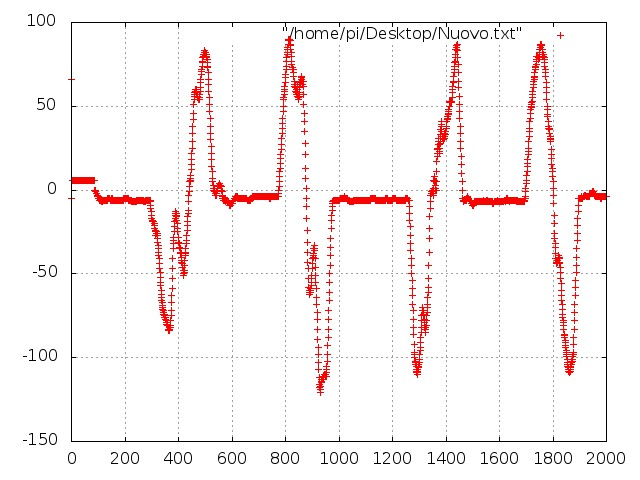Acceleration of an object moved from left to right, X4

I was trying to get the position of my accelerometer (relatively to its initial one) but googlein' around doesn't seem to be enough  this time. The concept is quite simple: since the acceleration is the first derivative of velocity, and position's second one, we could calculate the position of an object only by integrating (two times) the data from its acceleration. Ok, let's start from a good set of acceleration data. The graph above shows 2000 elements read out from the serial out of Arduino, with a rolling mean of 10 values. I'm trying to figure out why there are so many spikes to make it need 10 samples to get rid of them. Perhaps my "professional" solder process made some false-contact and the stirring of the circuits make them touch.

This is an example of this data loss (around the 925th sample) probably due to the phenomenon described above, or perhaps for the low quality of the contacts on the arduino side. Indeed I didn't use wires like these, but just a copper wire without its plastic cladding.
Anyway, let's do the dirty job. Since:

So, considering as a raw approximation a simple sum as the integrating function, we have that
1. velocity(i) = velocity(i-1) + acceleration (i)
2. position(i) = position (i-1) + velocity (i)
Whereas v(0)= p(0)= 0; For a first analysis I tried to implement the algorithm in Excel (offline, of course) and the partial results are show below. The blue line is the acceleration while the orange one is the velocity.Image 3

These are not from the same data as those in the first image, but the filtering is the same: a rolling mean of 10 values. The setup (Arduino + ADXL) is moved along the X-Axis for six times. Moreover, in this case I've already added a couple of further improvements. First, all the value below a threshold are thrown away to avoid the effect of the small vibrations. Second, since there could be an "offset" fixed value of static acceleration, this is calculated over a mean of 2048 samples and subtracted to all of the successive values.
Anyway we introduce an error at every step, which is integrated over time and so it keeps growing with the number of samples. If you click on the image 3 you can see how the velocity doesn't return to zero even between two movements!   The error in the estimation of the velocity brings to an even bigger error in the calculus of position: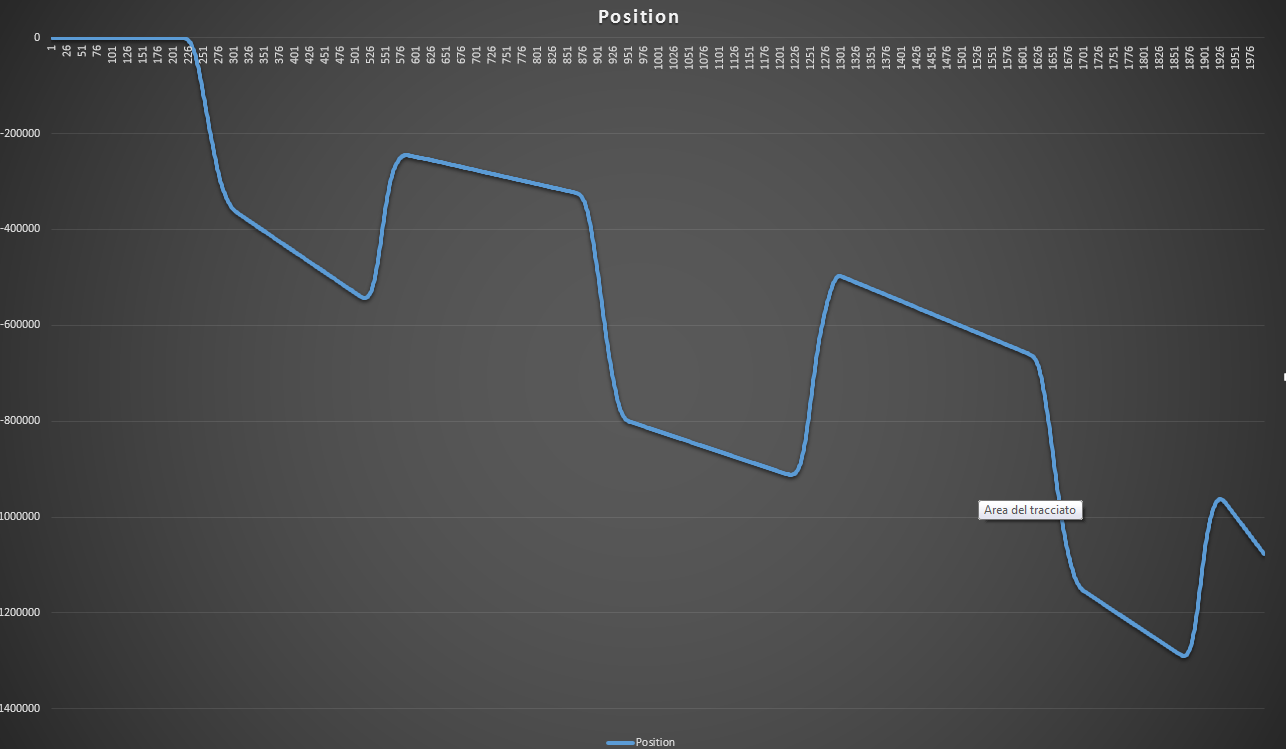Image 4
Even if the object was moving alternatively between two points, it seems moving away from them (the system is not stable). Ok, so far so good. It could not be so simple!  :)
Let's try to reduce this error. If you have any advice, question, or if you just want to insult me, do it in the comments below. See you soon.

## Wednesday, July 17, 2013

As many Arduino enthusiast, I bought a ADXL345 accelerometer from RS components and I set it up to communicate with my board in order to use it in a wider electronic project. I did want to share my experience. If you want to try the accelerometer yourself, you have two choises: buy the sparkfun BOB, which comes ready to be used, or buy the accelerometer and a couple of passive components from your preferred distributor and make your own Break Out Board.
I opted for the second one! ;)
Of course you'll need some hardware stuff, and the results will not be as good as the assembled one, but the satisfaction will be over 9000. For the breakout board you will need some bypass capacitors to solder as close as possible to the VCC pin of the SMD, which is a LGA. After some hours of super focused, high level, ultra professional layout design with EAGLE, and after a couple of iron stains on my jeans, the result is the following: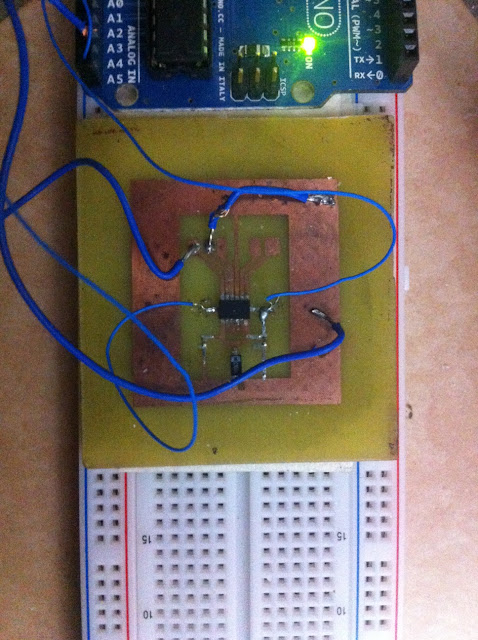ADXL345 on a DIY breakout board.

As you can see the result is quite obscene if compared to the red-fashioned ultra-small sparkfun edition, but this is a DIY and I love it!  In the image above the wire needed to provide power supply and I2C communication are already connected to my Arduino UNO. The second step of course was to google for some lines of code to read the accelerometer data. Internet is full of code for Arduino and this IC. Basically the code aims to:
1. Initialize the I2C communication setting the Arduino as Master & the IC as Slave.
2. Wake up the device from its low power mode (sleep mode)
3. Read out the X-Y-Z acceleration value.

I left out the function code for "readFrom" and "writeTo" to keep the post as compact as possible. If you need it, just leave a comment and I will send you the entire project. The image below is a snapshot from the IC's datashhet and it shows the meanings of the bits in the Power Control Register, whose addres is 0x2D. As you can see from the code above, we first reset the register writing to 0 every bit, and than we set to 1 the bit "Auto Sleep Mode" and "Measure". The latter is the one who let the device start measuring. Of course we could set the bit with a single instruction, but since this is kind a tutorial, I thought it was better to leave the three different tokens.
Next step: Calculate position from acceleration. If I reach 10 comments, I promise I'll do it in one day! ;)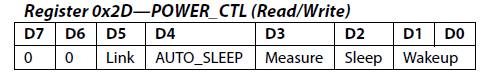ADXL345 POWER_CTL Register configuration byte